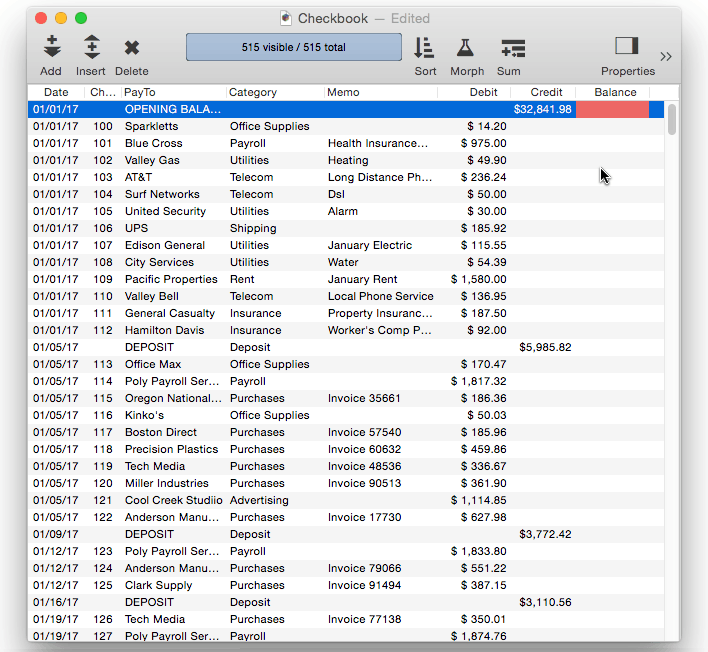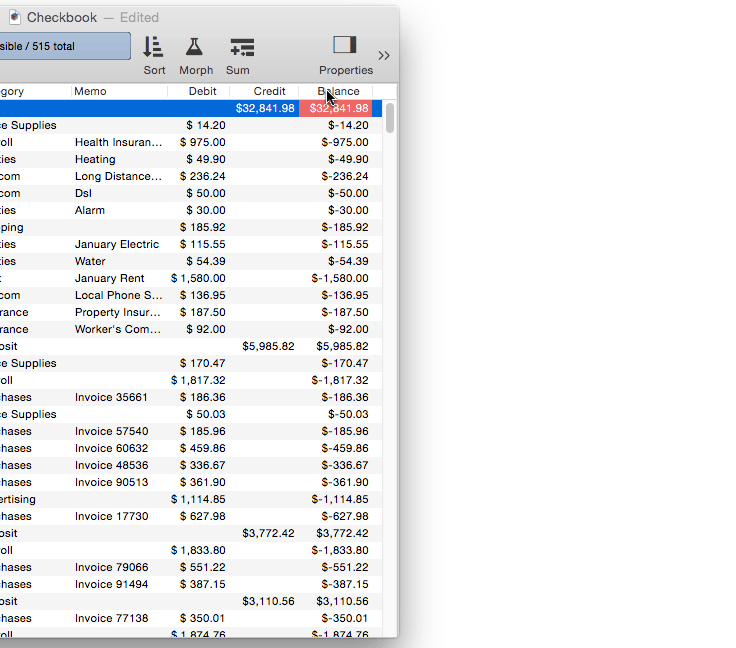Running Total is a special computation. Unlike the other summary calculations, Running Total modifies every data cell in the active field, not just the summary records. Like the Total computation, Running Total starts at the top of the database and adds up each data cell as it moves down the column. Running Total, however, replaces each data cell with the current total. The result is a field which contains the cumulative total at each point in the database. This is very useful for computing checking account balances, sales year to date, and other cumulative statistics.

If you use the Running Total command on your raw data, the raw data will be destroyed in the process of calculating the running total. We recommend that you avoid this problem by creating an extra field to hold the running total. You can use the Morph Field Dialog to copy the data into the field, and then use the Running Total command without disturbing the original raw data.

#### Using Running Total to Balance a Checkbook

Let’s take a look at how to balance a checkbook using the Running Total command. Start with an empty Balance field, and use the Morph Field command to calculate the how much the balance changes for each line (by subtracting Debit from Credit).Now use Running Total to calculate the balance after each transaction.You’ll need to repeat these steps when new transactions are added, or existing transactions changed. This process can be automated with a procedure.

``````field Balance
formulafill Credit-Debit
runningtotal
``````